# Surface Area Of Cylinders Worksheet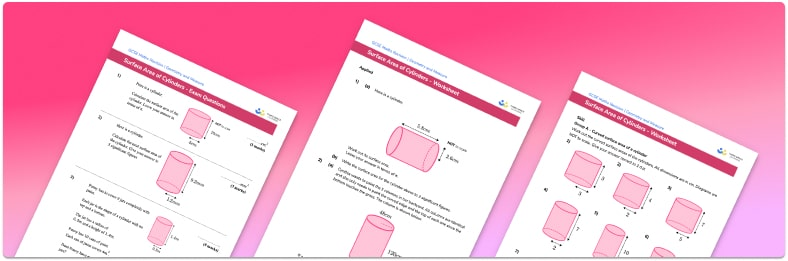• Section 1 of the surface area of cylinders worksheet contains 27 skills-based surface area of cylinders questions, in 3 groups to support differentiation
• Section 2 contains 4 applied surface area of cylinders questions with a mix of word problems and deeper problem solving questions
• Section 3 contains 3 foundation and higher level GCSE exam style surface area of cylinders questions
• Answers and a mark scheme for all surface area of cylinders questions are provided
• Questions follow variation theory with plenty of opportunities for students to work independently at their own level
• All questions created by fully qualified expert secondary maths teachers

Suitable for GCSE maths revision for AQA, OCR and Edexcel exam boards

• This field is for validation purposes and should be left unchanged.

You can unsubscribe at any time (each email we send will contain an easy way to unsubscribe). To find out more about how we use your data, see our privacy policy.

### Surface area of cylinders at a glance

Surface area is a measure of the total area of all of the faces of a 3D shape. A cylinder has 3 faces, two circular ends joined by a curved surface, which once unfolded is a rectangle. We know that the area of a circle is given by πr^2 , where r is the radius of the circle.

If we were to draw a net of a cylinder, the curved surface in the middle would open up to a rectangle with height h , the height of the cylinder, and length 2πr , the circumference of the circular base. For this reason, the curved surface area (also called lateral surface area) of a cylinder is given by 2πrh .

The total surface area of the cylinder is found by adding the areas of the three faces to give \text{Surface area} = 2π(r^2)+2πrh

Surface area of a cylinder is measured in square units such as cm^2 or mm^2 . We might be asked to give an answer in terms of pi or to round to a given number of decimal places or significant figures.

Looking forward, students can then progress to additional surface area worksheets and other geometry worksheets, for example a simplifying expressions worksheet or simultaneous equations worksheet.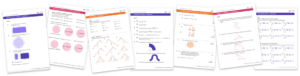For more teaching and learning support on Geometry our GCSE maths lessons provide step by step support for all GCSE maths concepts.

## Related worksheets

Surface Area Of A Triangular Prism Worksheet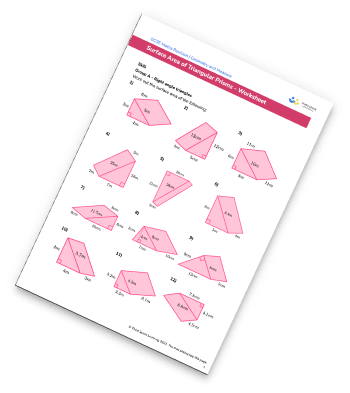Surface Area Of Cones Worksheet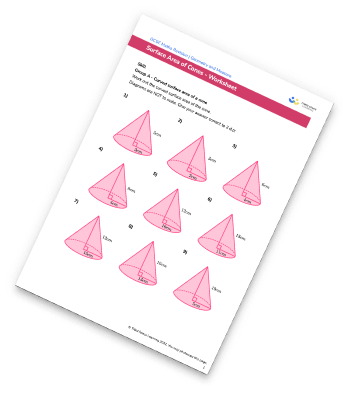Surface Area Of A Cuboid Worksheet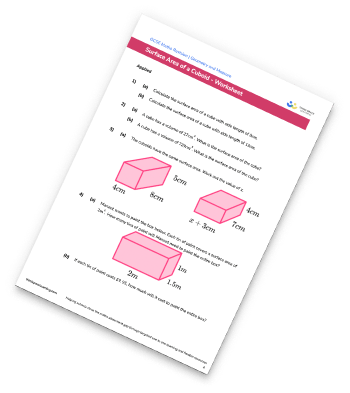Surface Area Of Prisms Worksheet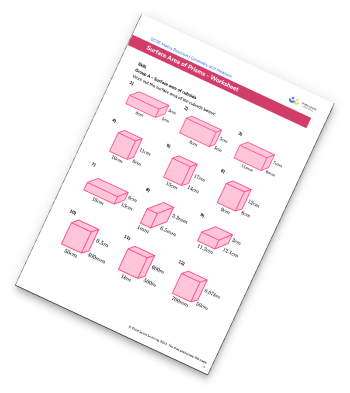## Do you have KS4 students who need more focused attention to succeed at GCSE?There will be students in your class who require individual attention to help them succeed in their maths GCSEs. In a class of 30, it’s not always easy to provide.

Help your students feel confident with exam-style questions and the strategies they’ll need to answer them correctly with our dedicated GCSE maths revision programme.

Lessons are selected to provide support where each student needs it most, and specially-trained GCSE maths tutors adapt the pitch and pace of each lesson. This ensures a personalised revision programme that raises grades and boosts confidence.

Find out more